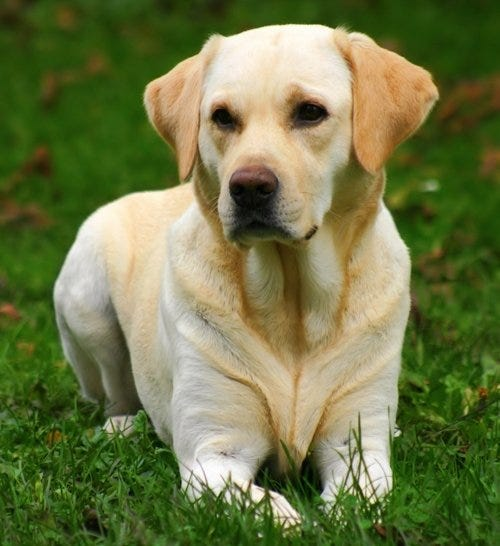# Dog Breed Classification using CNN

Original article was published by Opu chandraw on Deep Learning on Medium# Introduction:

The dog is a domesticated carnivoran of the family Canidae. It is part of the wolf-like canids and is the most widely abundant terrestrial carnivore.

I am in a Data Scientist nanodegree program in Udacity and taken over this project as my capstone project to predict a dog’s breed. In this project, I have built a pipeline that can be used within a web or mobile app to process real-world, user-supplied images. Given an image of a dog, my algorithm will identify an estimate of the canine’s breed. If supplied with an image of a human, the code will identify the resembling dog breed.

## Datasets:

3. We have a total of 13233 human images and 8351 different dog images with 133 different classes.

## Detect humans:

OpenCV provides many pre-trained face detectors, stored as XML files on Github. I have downloaded one of these detectors and stored it in the `haarcascades` directory. In the next code cell, I have demonstrated how to use this detector to find human faces in a sample image.

`import cv2 import matplotlib.pyplot as plt %matplotlib inline# extract pre-trained face detectorface_cascade = cv2.CascadeClassifier('haarcascades/haarcascade_frontalface_alt.xml')# load color (BGR) imageimg = cv2.imread(human_files)# convert BGR image to grayscalegray = cv2.cvtColor(img, cv2.COLOR_BGR2GRAY)# find faces in imagefaces = face_cascade.detectMultiScale(gray)#print number of faces detected in the imageimport torchimport torchvision.models as modelsprint(‘Number of faces detected:’, len(faces))# get bounding box for each detected facefor (x,y,w,h) in faces: #add bounding box to color image cv2.rectangle(img,(x,y),(x+w,y+h),(255,0,0),2)# convert BGR image to RGB for plottingcv_rgb = cv2.cvtColor(img, cv2.COLOR_BGR2RGB)# display the image, along with bounding boxplt.imshow(cv_rgb)plt.show()`

Human detector:

`def face_detector(img_path):    img = cv2.imread(img_path)    gray = cv2.cvtColor(img, cv2.COLOR_BGR2GRAY)    faces = face_cascade.detectMultiScale(gray)    return len(faces) > 0`

Dog detector:

`def dog_detector(img_path):    ## TODO: Complete the function.    index=VGG16_predict(img_path)    if index>=151 and index<=268:        return True     else:        return False`

## Create a CNN to Classify Dog Breeds (from Scratch):

Now that we have functions for detecting humans and dogs in images, we need a way to predict breed from images. In this step, I have created a CNN that classifies dog breeds.

Training images:

## Model Architecture:

`import torch.nn as nnimport torch.nn.functional as F# define the CNN architectureclass Net(nn.Module):    ### TODO: choose an architecture, and complete the class    def __init__(self):        super(Net, self).__init__()        ## Define layers of a CNN        # convolutional layer (sees 224x224x3 image tensor)        self.conv1 = nn.Conv2d(3, 32, 3, stride=2,padding=1)        self.conv2 = nn.Conv2d(32, 64, 3,stride=2, padding=1)        self.conv3 = nn.Conv2d(64, 128, 3, padding=1)        # max pooling layer        self.pool = nn.MaxPool2d(2, 2)        self.fc1 = nn.Linear(128*7*7, 1024)        # linear layer (500 -> 10)        self.fc2 = nn.Linear(1024, 512)        self.fc3 = nn.Linear(512,133)        # dropout layer (p=0.25)        self.dropout = nn.Dropout(0.25)    def forward(self, x):        ## Define forward behavior        x = self.pool(F.relu(self.conv1(x)))        x = self.pool(F.relu(self.conv2(x)))        x = self.pool(F.relu(self.conv3(x)))        # flatten image input        x = x.view(-1, 128*7*7)        # add dropout layer        x = self.dropout(x)        # add 1st hidden layer, with relu activation function        x = F.relu(self.fc1(x))        # add dropout layer        x = self.dropout(x)        # add 2nd hidden layer, with relu activation function        x = F.relu(self.fc2(x))        x = self.dropout(x)        x = F.relu(self.fc3(x))        return x# instantiate the CNNmodel_scratch = Net()# move tensors to GPU if CUDA is availableif use_cuda:    model_scratch.cuda()`

## Loss Function and Optimizer:

I have used CrossEntropyLoss() as a loss function and Stochastic Gradient Descent (SGD) as optimizers.

After training for 2 epochs I have found:

• Test Loss: 4.905558
• Accuracy of 12% in 836 test images.
• 101 out of 836 images were predicted correctly

## Create a CNN to Classify Dog Breeds (using Transfer Learning):

In this step, I have used transfer learning to create a CNN that can identify dog breed from images. In this case, I used resnet50 model architecture as a pre-trained network. The loss function and optimizer was the same as before.

After training it for 2 epochs I have got:

• Loss of 2.398314
• Accuracy of 66% in 836 test images
• 556 out of 836 images were predicted correctly

## The Algorithm for the app:

Algorithm:

`def run_app(img_path):    img = Image.open(img_path)    plt.imshow(img)    plt.show()    if dog_detector(img_path) is True:        prediction = predict_breed_transfer(model_transfer, class_names,img_path)        print("Dog Detected!\nIt's a {0}".format(prediction))      elif face_detector(img_path) > 0:        prediction = predict_breed_transfer(model_transfer, class_names,img_path)        print("It's a human!\nIn case of a dog it would be {0}".format(prediction))    else:        print("It's neither dog or human")`

In this step, I wrote an algorithm that accepts a file path to an image and first determines whether the image contains a human, dog, or neither. Then,

• if a dog is detected in the image, return the predicted breed.
• if a human is detected in the image, return the resembling dog breed.
• if neither is detected in the image, provide an output that indicates an error.

## Conclusion:

As my testing accuracy was not so good, with that considering it’s performing better. Three possible points for improvement,

1. Need to train the model much longer
2. More hidden layers can be added to detect more complex features
3. By tuning the optimizer